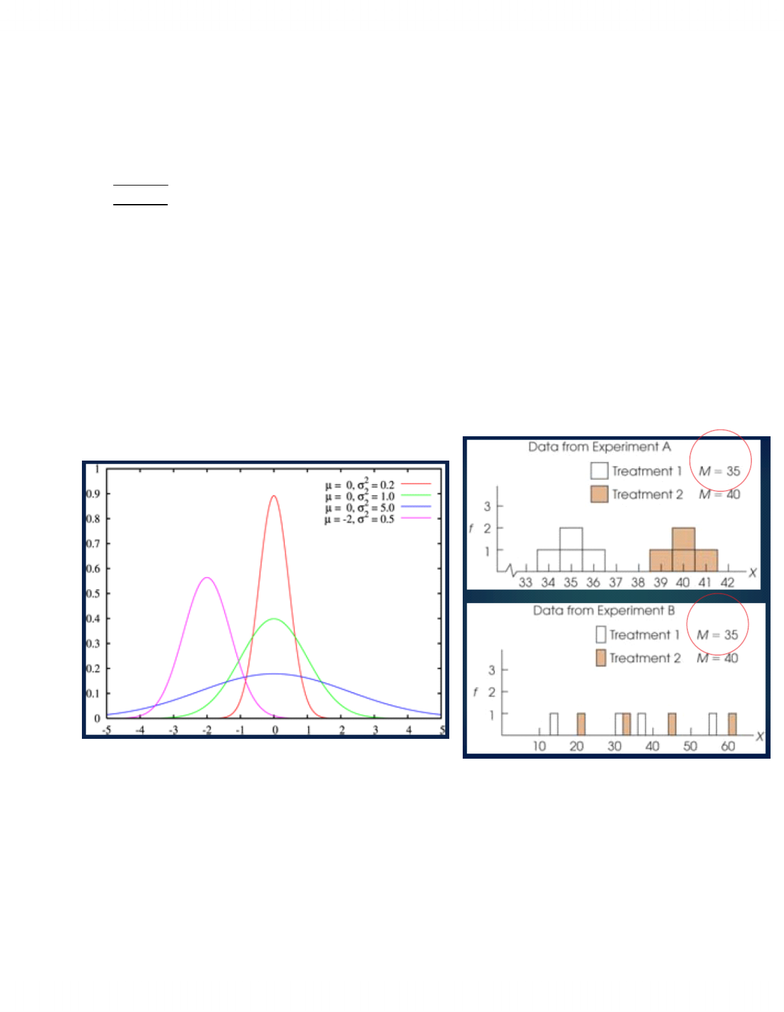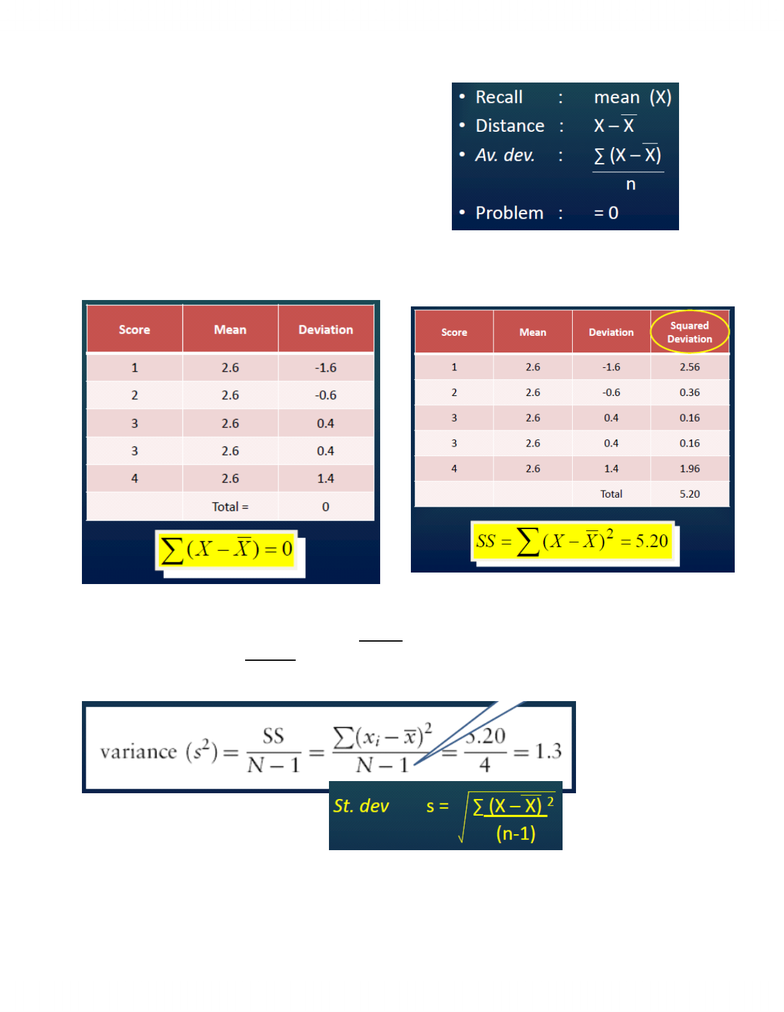# PSYC 2530 Lecture Notes - Central Tendency, Statistical Model, Standard Deviation

15 views6 pagesBeyond typical value --> typical dispersion
-
-
Decrease in predictability
Decrease in homogeneity
Increase in Heterogeneity
Increase in variability =
Problem:
-
Goal : measure spread of scores in a distribution
-
Descriptive : degree to which scores in a sample are spread out or clustered
-
Small V = scores clustered --> any score gives a good representation of the population
Large V = scores widely spread --> a few extreme scores can give a distorted picture of the general
population
Inferential : how accurately any one score or sample represents the population
-
Variability
Range
Standard deviation & variance
Variability can be measured by the
-
Between scores, variability measures distance
-
Measuring Variability
Total distance spanned by sample scores
-
Only reflects extremes (typical = middle)
"rough" (approximation, unrefined)
-
Range
Ch. 4 - Variability
Wednesday, September 19, 2012
3:06 PM
Lecture Notes Page 1
Unlock document

This preview shows pages 1-2 of the document.
Unlock all 6 pages and 3 million more documents.Only reflects extremes (typical = middle)
Unstable
Fast and easy to calculate
-
Value (e.g., winter temperature)
-
e.g., 7 +- 2, "more or less"
"Normal", "common", "typical", dispersion
-
Try average distance between scores & mean
-
Standard Deviation
Sum deviations for each score (value -- mean)
-
Calculate "average" distance
Sum of Squares (SS): good measure of overall variability, but dependent on the number of scores
-
So, we calculate the average variability: SS/(n-1)
-
This value is called the variance (s2)
-
Variance
Sample variance : s2
-
Population variance : σ2
-
Standard Deviation
Standard Deviation: calculation
Sum of squared deviations score to mean?
Note: N-1, not N
Lecture Notes Page 2
Unlock document

This preview shows pages 1-2 of the document.
Unlock all 6 pages and 3 million more documents.